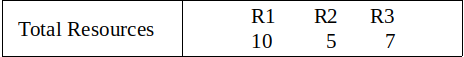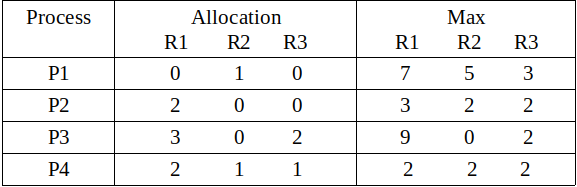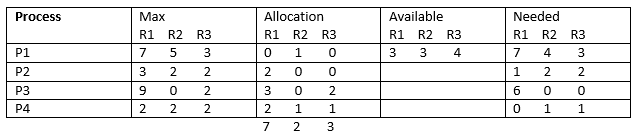# Banker’s Algorithm in Operating System

• Difficulty Level : Medium
• Last Updated : 15 Jun, 2021

Prerequisite – Resource Allocation Graph (RAG), Banker’s Algorithm, Program for Banker’s Algorithm
Banker’s Algorithm is a resource allocation and deadlock avoidance algorithm. This algorithm test for safety simulating the allocation for predetermined maximum possible amounts of all resources, then makes an “s-state” check to test for possible activities, before deciding whether allocation should be allowed to continue.

In simple terms, it checks if allocation of any resource will lead to deadlock or not, OR is it safe to allocate a resource to a process and if not then resource is not allocated to that process. Determining a safe sequence(even if there is only 1) will assure that system will not go into deadlock.

Banker’s algorithm is generally used to find if a safe sequence exist or not. But here we will determine the total number of safe sequences and print all safe sequences.

The data structure used are:

• Available vector
• Max Matrix
• Allocation Matrix
• Need Matrix

Example:
Input:```Output: Safe sequences are:
P2--> P4--> P1--> P3
P2--> P4--> P3--> P1
P4--> P2--> P1--> P3
P4--> P2--> P3--> P1

There are total 4 safe-sequences ```

Explanation:
Total resources are R1 = 10, R2 = 5, R3 = 7 and allocated resources are R1 = (0+2+3+2 =) 7, R2 = (1+0+0+1 =) 2, R3 = (0+0+2+1 =) 3. Therefore, remaining resources are R1 = (10 – 7 =) 3, R2 = (5 – 2 =) 3, R3 = (7 – 3 =) 4.
Remaining available = Total resources – allocated resources
and
Remaining need = max – allocatedSo, we can start from either P2 or P4. We can not satisfy remaining need from available resources of either P1 or P3 in first or second attempt step of Banker’s algorithm. There are only four possible safe sequences. These are :
P2–> P4–> P1–> P3
P2–> P4–> P3–> P1
P4–> P2–> P1–> P3
P4–> P2–> P3–> P1

Implementation:

.

Output:

```Safe sequences are:
P2--> P4--> P1--> P3
P2--> P4--> P3--> P1
P4--> P2--> P1--> P3
P4--> P2--> P3--> P1

There are total 4 safe-sequences```

My Personal Notes arrow_drop_up